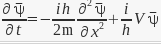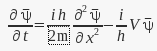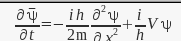# Schrodinger equation in terms of complex conjugate

QuantumDuality
I know there's a similar post, but i didn't understand it. Why the derivative respect to t in terms of the complex conjugate of ψ is:instead of being the original S.E in terms of ψ*or the equation in terms of ψ with the signs swappedGold Member
One is simply applying conjugation to the equation as a whole. This yields an equation which is valid provided the original is valid. Some c-number conjugation rules that may be helpful are,

1. ##(a b)^\ast = a^\ast b^\ast##
2. ##(a + b)^\ast = a^\ast + b^\ast##
3. ##(i)^\ast = -i##
4. ##(\frac{\partial \psi}{\partial t})^\ast = \frac{\partial \bar\psi}{\partial t}##

QuantumDuality
I got it!. I always forget to use the product rule, Thanks